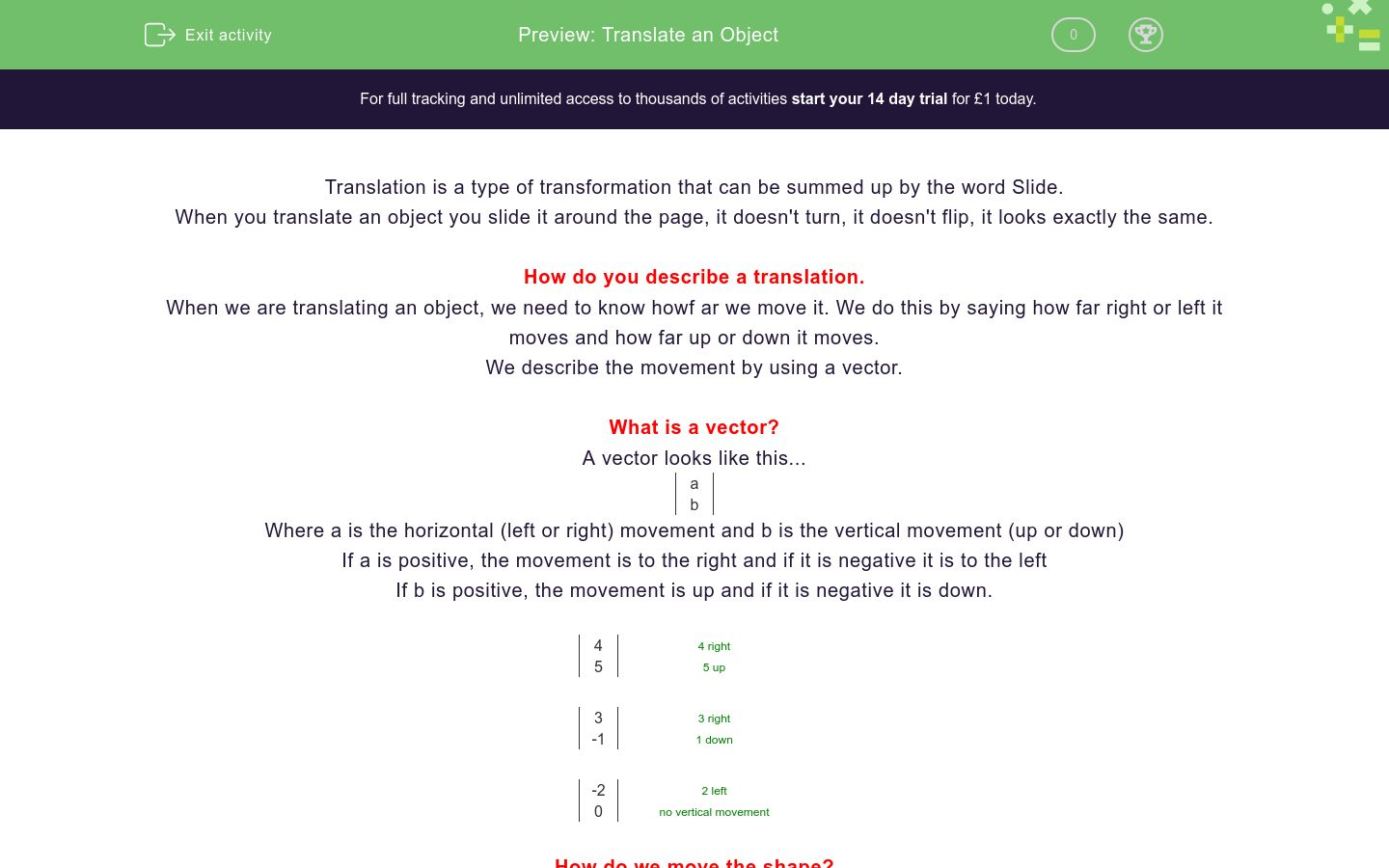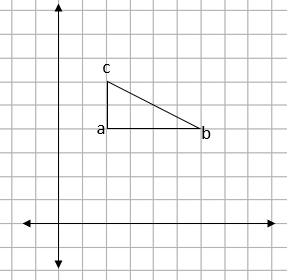# Translate an Object

In this worksheet, students practise translating an object.Key stage:  KS 4

GCSE Subjects:   Maths

GCSE Boards:   AQA, Eduqas, Pearson Edexcel, OCR

Curriculum topic:   Geometry and Measures, Congruence and Similarity

Curriculum subtopic:   Properties and Constructions, Plane Vector Geometry

Difficulty level:### QUESTION 1 of 10

Translation is a type of transformation that can be summed up by the word Slide.

When you translate an object you slide it around the page, it doesn't turn, it doesn't flip, it looks exactly the same.

How do you describe a translation.

When we are translating an object, we need to know howf ar we move it. We do this by saying how far right or left it moves and how far up or down it moves.

We describe the movement by using a vector.

What is a vector?

A vector looks like this...

 a b

Where a is the horizontal (left or right) movement and b is the vertical movement (up or down)

If a is positive, the movement is to the right and if it is negative it is to the left

If b is positive, the movement is up and if it is negative it is down.

 4 4 right 5 5 up

 3 3 right -1 1 down

 -2 2 left 0 no vertical movement

How do we move the shape?

The key thing about translations is that every point moves the same distance so all we have to do is to translate each corner of the shape by the same amount and then join up the points.

Example:

 Translate shape a by the vector... 1 -4

This vector means we have to move the shape 1 to the right and 4 down.Which word can we use to describe translations?

Flip

Turn

Slide

A translation is always described using a vector of the form...

 a b
Flip

Turn

Slide

What translation does this vector give?

 2 3

What translation does this vector give?

 3 -2

Moving 5 to the right and 3 up will give the vector...

 a b
 Value a b

Moving 2 to the left and then 1 down

 a b
 Value a bThe triangle ABC is translated by the vector

 3 2

Find the coordinate of b'

Give your answer in the form (a,b). Make sure you don't put any spaces in the answer.The triangle ABC is translated by the vector

 -1 -2

Find the coordinate of c'

Give your answer in the form (a,b). Make sure you don't put any spaces in the answer.A shape is translated by the vector

 2 -3

Which two of the shapes were involved in this translation?

A

B

C

D

EA shape is translated by the vector

 5 0

Which two of the shapes were involved in this translation?

A

B

C

D

E

• Question 1

Which word can we use to describe translations?

Slide
EDDIE SAYS
Each of the transfornations has a word that describes its use. Flip is a reflection Turn is a rotation Slide is a translation
• Question 2

A translation is always described using a vector of the form...

 a b
EDDIE SAYS
Vectors can be remembered by the same method as coordinates. 'Along the corridor and up the stairs' Pulling out the key words gives 'Along, Up' This then becomes 'Horizontal, Vertical'
• Question 3

What translation does this vector give?

 2 3
EDDIE SAYS
Positive is up and right, negative is left and down.
• Question 4

What translation does this vector give?

 3 -2
EDDIE SAYS
Positive is up and right, negative is left and down.
• Question 5

Moving 5 to the right and 3 up will give the vector...

 a b
 Value a b
EDDIE SAYS
a is the horizontal movement and is to the right (+ve) b is the vertical movement and is up (+ve)
• Question 6

Moving 2 to the left and then 1 down

 a b
 Value a b
EDDIE SAYS
a is the horizontal movement and is to the left (-ve) b is the vertical movement and is down (-ve)
• Question 7The triangle ABC is translated by the vector

 3 2

Find the coordinate of b'

Give your answer in the form (a,b). Make sure you don't put any spaces in the answer.

(9,6)
EDDIE SAYS
The point B is currently at (6,4) The vector means 3 to the right and 2 up.
• Question 8The triangle ABC is translated by the vector

 -1 -2

Find the coordinate of c'

Give your answer in the form (a,b). Make sure you don't put any spaces in the answer.

(1,4)
EDDIE SAYS
The point B is currently at (2,6) The vector means 1 to the left and 2 down. Where does that get us to?
• Question 9A shape is translated by the vector

 2 -3

Which two of the shapes were involved in this translation?

C
D
EDDIE SAYS
For this one, we need to be looking at which ones have a horizontal difference of 2 and a vertical difference of 3. The only ones that fit this are C as the original and D as the translated shape
• Question 10A shape is translated by the vector

 5 0

Which two of the shapes were involved in this translation?

B
D
EDDIE SAYS
For this one, we can notice that the vertical difference is 0, this means they must be in line horizontally. The only ones that fit this are B as the original and D as the translated shape
---- OR ----